# Finiteness of lattice points on varieties F(y) = F(g(𝕏)) + r(𝕏) over imaginary quadratic fields

Lukasz Nizio
Notes on Number Theory and Discrete Mathematics
Print ISSN 1310–5132, Online ISSN 2367–8275
Volume 27, 2021, Number 1, Pages 76—90
DOI: 10.7546/nntdm.2021.27.1.76-90

## Details

### Authors and affiliations

Lukasz NizioFaculty of Mathematics and Computer Science, Adam Mickiewicz University
Uniwersytetu Poznanskiego 4, 61-614 Poznan, Poland

### Abstract

We construct affine varieties over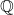and imaginary quadratic number fields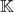with a finite number of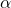-lattice points for a fixed, wheredenotes the ring of algebraic integers of. These varieties arise from equations of the form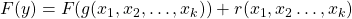, whereis a rational function,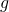and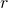are polynomials over, and the degree ofis relatively small. We also give an example of an affine variety of dimension two, with a finite number of algebraic integral points. This variety is defined over the cyclotomic field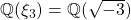.

### Keywords

• Diophantine equations
• Algebraic integral points
• Higher dimension affine varieties

• 11D45
• 11G35

### References

1. Arimoto, K., & Hirano, Y. (2019). A generalization of LLL lattice basis reduction over imaginary quadratic fields. Scientiae Mathematicae Japonicae, 82(1), 1–6.
2. Bashenov, A., Bilu, M., Bilu, Y. F., & Rath, P. (2015). Rational points on analytic varieties. EMS Surveys in Mathematical Sciences, 2, 109–130.
3. Erdős, P. (1939). Note on products of consecutive integers. Journal of the London Mathematical Society, 14(3), 194–198.
4. Erdős, P., & Selfridge, J. L. (1975). The product of consecutive integers is never a power. Illinois Journal of Mathematics, 19(2), 292–301.
5. Hardy, G. H., & Wright, E. M. (1979). An Introduction to the Theory of Numbers. University Press Oxford, 7–8.
6. Hindry, M., & Silverman, J. H. (2000). Diophantine Geometry: An Introduction. Springer-Verlag, New York, 299–366.
7. Silverman, J. H. (2009). The Arithmetic of Elliptic Curves. Springer-Verlag, New York.
8. Wiles, A. (1995). Modular elliptic curves and Fermat’s Last Theorem. Annals of Mathematics, 141(3), 443–551.

## Cite this paper

Nizio, L. (2021). Finiteness of lattice points on varieties F(y) = F(g(𝕏)) + r(𝕏) over imaginary quadratic fields. Notes on Number Theory and Discrete Mathematics, 27(1), 76-90, doi: 10.7546/nntdm.2021.27.1.76-90.